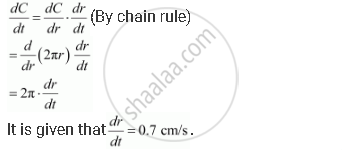Share

# The Radius of a Circle is Increasing at the Rate of 0.7 Cm/S. What is the Rate of Increase of Its Circumference? - CBSE (Science) Class 12 - Mathematics

ConceptRate of Change of Bodies Or Quantities

#### Question

The radius of a circle is increasing at the rate of 0.7 cm/s. What is the rate of increase of its circumference?

#### Solution

The circumference of a circle (C) with radius (r) is given by

C = 2πr.

Therefore, the rate of change of circumference (C) with respect to time (t) is given by,Hence, the rate of increase of the circumference  is 2pi(0.7) = 1.4 pi "cm/s"

Is there an error in this question or solution?

#### APPEARS IN

NCERT Solution for Mathematics Textbook for Class 12 (2018 to Current)
Chapter 6: Application of Derivatives
Q: 6 | Page no. 198

#### Video TutorialsVIEW ALL 

Solution The Radius of a Circle is Increasing at the Rate of 0.7 Cm/S. What is the Rate of Increase of Its Circumference? Concept: Rate of Change of Bodies Or Quantities.
S